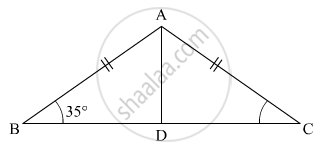# Abc is an Isosceles Triangle Such that Ab = Ac and Ad is the Median to Base Bc. Then, ∠Bad = - Mathematics

MCQ

ABC is an isosceles triangle such that AB = AC and AD is the median to base BC. Then, ∠BAD =•  55°

•  70°

• 35°

• 110°

#### Solution

It is given that ∠B = 35°, AB=AC and Ad is the median of BCWe know that in isosceles triangle the median from he vertex to the unequal side divides it into two equal part at right angle.

Therefore,

∠B = ∠ADB + ∠A= 180° (Property of triangle)

35° + 90° +∠A = 180°

∠A = 180° - 125°

∠A = 55°

Concept: Congruence of Triangles
Is there an error in this question or solution?

#### APPEARS IN

RD Sharma Mathematics for Class 9
Chapter 12 Congruent Triangles
Exercise 12.8 | Q 18 | Page 87
Share